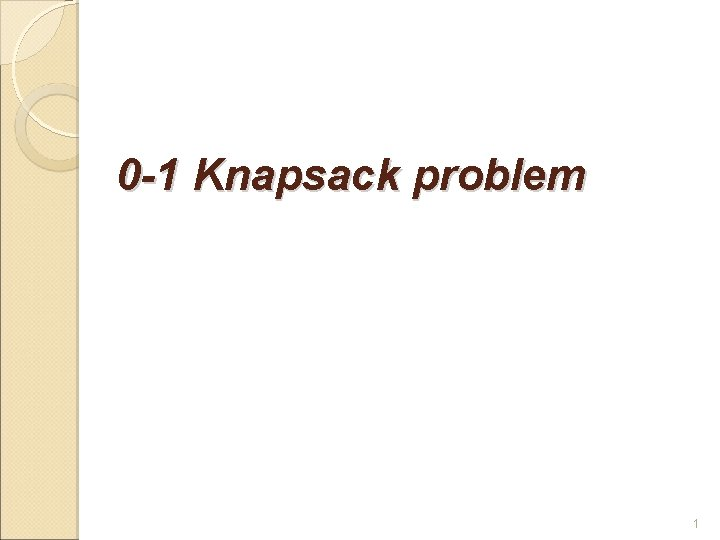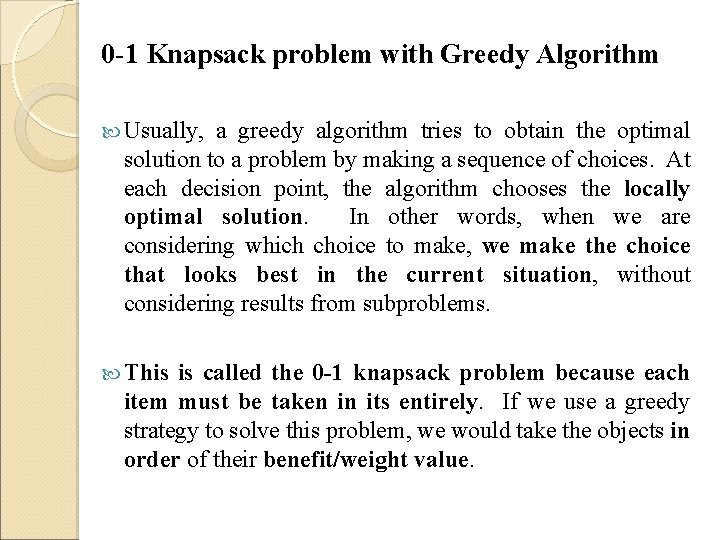# 0 1 Knapsack problem 1 0 1 Knapsack

• Slides: 50 -1 Knapsack problem 10 -1 Knapsack problem with Greedy Algorithm Usually, a greedy algorithm tries to obtain the optimal solution to a problem by making a sequence of choices. At each decision point, the algorithm chooses the locally optimal solution. In other words, when we are considering which choice to make, we make the choice that looks best in the current situation, without considering results from subproblems. This is called the 0 -1 knapsack problem because each item must be taken in its entirely. If we use a greedy strategy to solve this problem, we would take the objects in order of their benefit/weight value.The Greedy method does not work for the 0 -1 Knapsack Problem.0 -1 Knapsack problem with Evolutionary Algorithm A set of n items and a set of k knapsacks are considered, for each item are know: Pi, j – profit of item j according to knapsack i; Wi, j – weight of item j according to knapsack i. The capacity of each knapsack i is ci. The problem is to find a vector x = (x 1, x 2, …, xn) ∈ {0, 1}n such that the capacity constraints are satisfied and for which f(x) = (f 1(x), f 2(x), …, fk(x)) is maximum, where and xj = 1 if the item j is selected and xj = 0 otherwise.The proposed algorithm can be described as follows: Each individual consists of a string of zero and one. The value one for the position j of the chromosome means that the item j was selected. The algorithm starts with a population of such individuals. For each chromosome the total items weight for each knapsack is computed. If there are knapsacks for which the allowed capacity is overdone the items beginning with one for which the proportion utility/weight is lowest are eliminated. This process continues until there are no knapsacks for which the capacity is overdone. All nondominated solutions are founded. The ε-dominance concept is used. Selection is applied by randomly chosing of two solutions. Convex crossover operator and mutation are applied. Two offspring are obtained. For each offspring the procedure for eliminating items if the capacity of one of knapsacks is overhead is used. The offspring enter the population of the new generation.